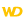• Limitations
• Coordinates
• Solid figure
• Drawing in PHP
• Drawing in Browser code
WINDEVWEBDEVWINDEV MobileOthers<WDPic variable>.DrawChord (Function)
In french: <Variable WDPic>.DessineCorde
Draws the chord of a circle (intersection between an ellipse and a straight line) in a variable of type WDPic.
Example
MyImage is WDPic = "Test.gif"
// In a WDPic variable, draw the chord of a circle whose
// background is light yellow and whose border is light blue
MyImage.DrawChord(10, 10, 50, 50, 20, 20, 40, 50, LightYellow, LightBlue)

IMG_MyDrawing = MyImage
Syntax
<WDPic image>.DrawChord(<X1> , <Y1> , <X2> , <Y2> , <X3> , <Y3> , <X4> , <Y4> [, <Background color> [, <Line color>]])
<WDPic image>: WDPic variable
Name of the WDPic variable to be used. Only the background layer will be handled.
<X1>: Integer
X-coordinate of the upper-left corner of the rectangle containing the circle. These coordinates are expressed in pixels.
<Y1>: Integer
Y-coordinate of the upper-left corner of the rectangle containing the circle. These coordinates are expressed in pixels.
<X2>: Integer
X-coordinate of the lower-right corner of the rectangle containing the circle. These coordinates are expressed in pixels.
<Y2>: Integer
Y-coordinate of the lower-right corner of the rectangle containing the circle. These coordinates are expressed in pixels.
<X3>: Integer
X-coordinate of the start point of the chord. These coordinates are expressed in pixels.
<Y3>: Integer
Y-coordinate of the start point of the chord. These coordinates are expressed in pixels.
<X4>: Integer
X-coordinate of the end point of the chord. These coordinates are expressed in pixels.
<Y4>: Integer
Y-coordinate of the end point of the chord. These coordinates are expressed in pixels.
<Background color>: Integer or constant (optional)
Background color of the chord. This color can correspond to:
• an RGB color (returned by RGB),
• an HSL color (returned by HSL),
• a WLanguage preset color,
•a variable of type Color.
If this parameter is not specified, the background color:
<Line color>: Integer or constant (optional)
Line color for the circle chord. This color can correspond to:
• an RGB color (returned by RGB),
• an HSL color (returned by HSL),
• a WLanguage preset color,
•a variable of type Color.
If this parameter is not specified, the line color:
Remarks

Limitations

• <WDPic variable>.DrawChord is not available in anti-aliasing mode (for more details, see the help about <WDPic variable>.ChangeMode).
• <WDPic variable>.DrawChord is not available for a drawing with management of alpha channel (for more details, see the help about <WDPic variable>.StartDrawing). This function has no effect.

Coordinates

If the start and end points are not located on the outline of the circle (or on the outline of the ellipse), the point taken into account is the intersection between the circle and the line that joins the specified point and the center of the rectangle (which means the center of the circle).The coordinates are specified with respect to the upper-left corner of the Image control (coordinates set to (0,0)).

Solid figure

<WDPic variable>.DrawChord can only be used to draw a chord: outline only or chord area. To draw a solid figure (portion defined by the center of the circle and the two points on the circle), use <WDPic variable>.DrawSlice.
Related Examples:Unit examples (WINDEV): The drawing functions [ + ] Using the main drawing functions of WINDEV to:- Initialize an Image control for drawing- Draw simple shapes- Write a text into a drawing- Change the color in a drawing
Business / UI classification: Neutral code
Component: wd280pnt.dll
Minimum version required
• Version 25